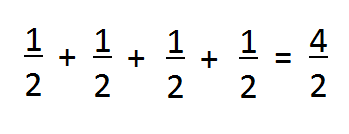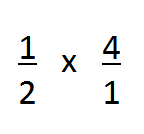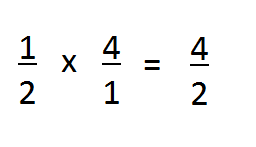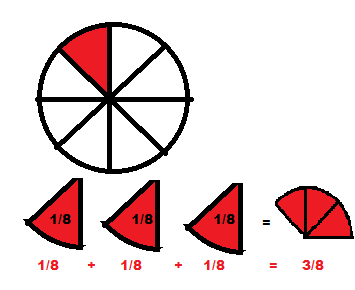# Multiplying Fractions by Whole Numbers: Lesson for Kids

An error occurred trying to load this video.

Try refreshing the page, or contact customer support.

Coming up next: How to Simplify Fractions: Lesson for Kids

### You're on a roll. Keep up the good work!

Replay
Your next lesson will play in 10 seconds
• 0:04 Fraction Basics
• 0:43 Fractions & Whole Numbers
• 1:32 Sharing A Pizza
• 2:02 Calculate Age
• 2:32 Lesson Summary
Save Save

Want to watch this again later?

Timeline
Autoplay
Autoplay
Speed Speed

#### Recommended Lessons and Courses for You

Lesson Transcript
Instructor: Dina Albert
In this lesson, you will learn how to multiply a fraction by a whole number. You'll work through real-world examples where it's necessary to multiply fractions by whole numbers, then you can test yourself with a short quiz.

## Fraction Basics

Fractions make up a lot of the world around us. They're used in cooking, building structures, drawing, and even shopping. So it's important to know how to multiply fractions with not only other fractions, but also whole numbers.

But before we dive into multiplying fractions by whole numbers, let's review a few important basics about fractions:

• When adding fractions with common denominators, add the numbers on top, which are known as numerators. Leave the denominator (the number on the bottom) the same.
• When multiplying fractions, multiply all the numerators going across and then all the denominators going across.
• Improper fractions are fractions where the numerator is bigger than the denominator.

## Fractions & Whole Numbers

Let's say you are baking cookies and the recipe requires 1/2 cup of sugar for one batch. But you want to make 4 batches. So you would need 4 times the amount of sugar. To figure out how much sugar you need altogether, you could add 1/2 cup 4 times:A faster method to find this same answer is to turn the whole number (4) into an improper fraction, then multiply it by the 1/2 cup of sugar. To turn a whole number into a fraction, simply make the whole number the numerator, and make 1 the denominator. So, in fraction form, 4 is represented as 4/1. Now, multiply:When multiplying fractions, multiply all the numerators going across and then all the denominators going across:You can simplify the improper fraction into a whole number by dividing the numerator by the denominator: 4 / 2 = 2. You need 2 cups of sugar.

## Sharing a Pizza

Let's look at another example. Say you've made a pizza and cut it into 8 slices. You're giving one slice each to three friends. How much pizza will be eaten all together?

We could solve this problem by adding 1/8 three times:To unlock this lesson you must be a Study.com Member.

### Register to view this lesson

Are you a student or a teacher?

#### See for yourself why 30 million people use Study.com

##### Become a Study.com member and start learning now.
Back
What teachers are saying about Study.com

### Earning College Credit

Did you know… We have over 200 college courses that prepare you to earn credit by exam that is accepted by over 1,500 colleges and universities. You can test out of the first two years of college and save thousands off your degree. Anyone can earn credit-by-exam regardless of age or education level.